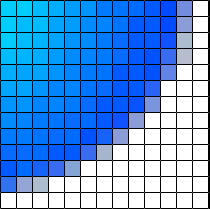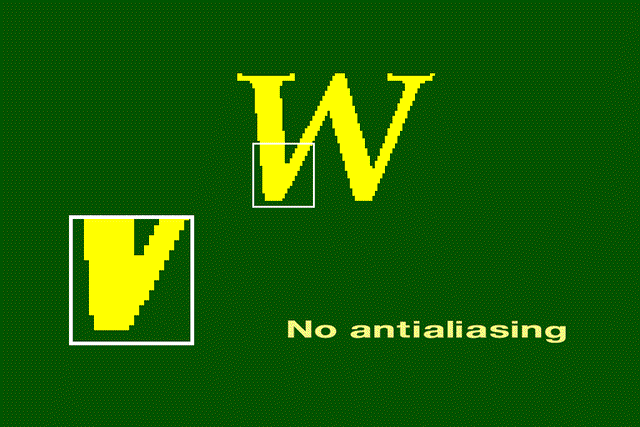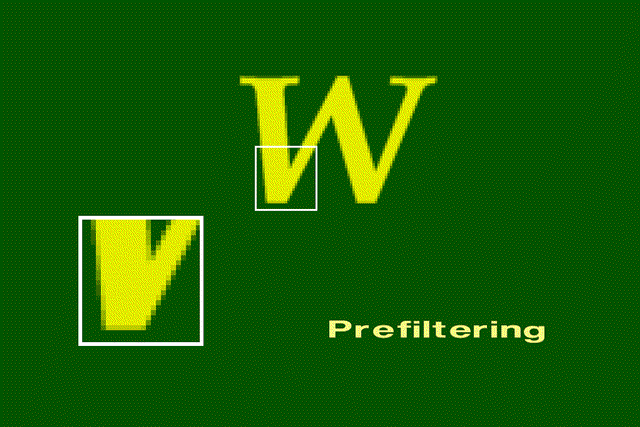## Antialiasing strategies

Antialiasing methods were developed to combat the effects of aliasing.

There are three main classes of antialiasing algorithms.

• As aliasing problem is due to low resolution, one easy solution is to increase the resolution , causing sample points to occur more frequently. This increases the cost of image production.

• The image is created at high resolution and then digitally filtered. This method is called supersampling or postfiltering and eliminates high frequencies which are the source of aliases.

• The image can be calculated by considering the intensities over a particular region. This is called prefiltering.

• #### Prefiltering.

Prefiltering methods treat a pixel as an area, and compute pixel color based on the overlap of the scene's objects with a pixel's area. These techniques compute the shades of gray based on how much of a pixel's area is covered by a object.
For example, a modification to Bresenham's algorithm was developed by Pitteway and Watkinson. In this algorithm, each pixel is given an intensity depending on the area of overlap of the pixel and the line. So, due to the blurring effect along the line edges, the effect of antialiasing is not very prominent, although it still exists.
Prefiltering thus amounts to sampling the shape of the object very densely within a pixel region. For shapes other than polygons, this can be very computationally intensive.#### Original Image

Without antialiasing, the jaggies are harshly evident.#### Prefiltered image

.
Along the character's border, the colors are a mixture of the foreground and background colors.#### Postfiltering.

Supersampling or postfiltering is the process by which aliasing effects in graphics are reduced by increasing the frequency of the sampling grid and then averaging the results down. This process means calculating a virtual image at a higher spatial resolution than the frame store resolution and then averaging down to the final resolution. It is called postfiltering as the filtering is carried out after sampling.

There are two drawbacks to this method

• The drawback is that there is a technical and economic limit for increasing the resolution of the virtual image.

• Since the frequency of images can extend to infinity, it just reduces aliasing by raising the Nyquist limit - shift the effect of the frequency spectrum.
• Supersampling is basically a three stage process.

• A continuous image I(x,y) is sampled at n times the final resolution. The image is calculated at n times the frame resolution. This is a virtual image.

• The virtual image is then lowpass filtered

• The filtered image is then resampled at the final frame resolution.
• #### Algorithm for supersampling

• To generate the origial image, we need to consider a region in the virtual image. The extent of that region determines the regions involved in the lowpass operation. This process is called convolution.

• After we obtain the virtual image which is at a higher resolution, the pixels of the final image are located over superpixels in the virtual image. To calculate the value of the final image at (Si,Sj), we place the filter over the superimage and compute the sum of the filter weights and the surrounding pixels. An adjacent pixel of the final image is calculated by moving the filter S superpixels to the right. Thus the step size is same as the scale factor between the real and the virtual image.
• Filters combine samples to compute a pixel's color. The weighted filter shown on the slide combines nine samples taken from inside a pixel's boundary. Each sample is multiplied by its corresponding weight and the products are summed to produce a weighted average, which is used as the pixel color. In this filter, the center sample has the most influence. The other type of filter is an unweighted filter. In an unweighted filter, each sample has equal influence in determining the pixel's color. In other words, an unweighted filter computes an unweighted average.
• The spatial extent of the filter determines the cutoff frequency. The wider the filter, the lower is the cutoff frequency and the more blurred is the image.
• The options available in supersampling are

• The value of S - scaling factor between the virtual and the real images.

• The choice of the extents and the weights of the filter
• As far the first factor is concerned, higher the value, the better the result is going to be. The compromise to be made is the high storage cost.

• It is not a context sensitive technique and thereby results in a lot of wasteful computations.

• A few examples of supersampling

There are two other categories of sampling

• Stochastic Sampling

•Back to the main screen